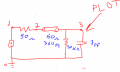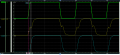# understanding reflection logic of this simple circuit

#### yef smith

Joined Aug 2, 2020
293
Hello,if we look at the circuit bellow then we see gamma_g=(50-50)/100=0 gamma_load=(10000-1)/10001=1
at first we have a voltage divider to V=5 and 2.5 is sent to the load.
if the load was only a resistor then i expect 2.5*1 to go toward the generator and never come back because gamma_g=0
but there is a capacitor and resistor on the load.
I cant imagine the coefficient logic in constructing the waveform#### Deleted member 115935

Joined Dec 31, 1969
0

#### yef smith

Joined Aug 2, 2020
293

#### Delta Prime

Joined Nov 15, 2019
1,161
This is a test right?